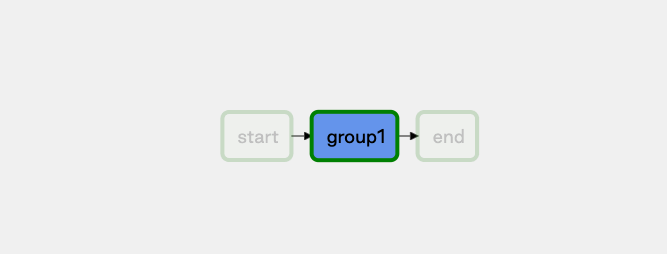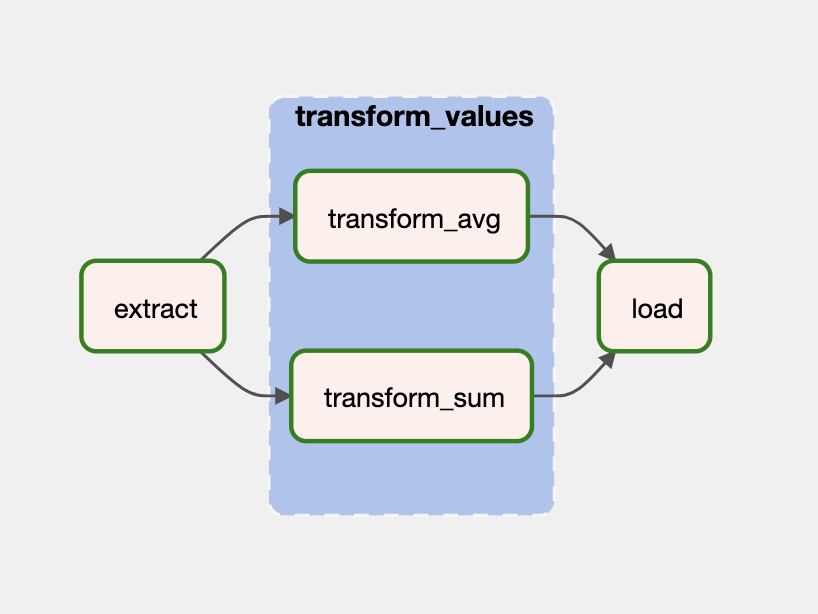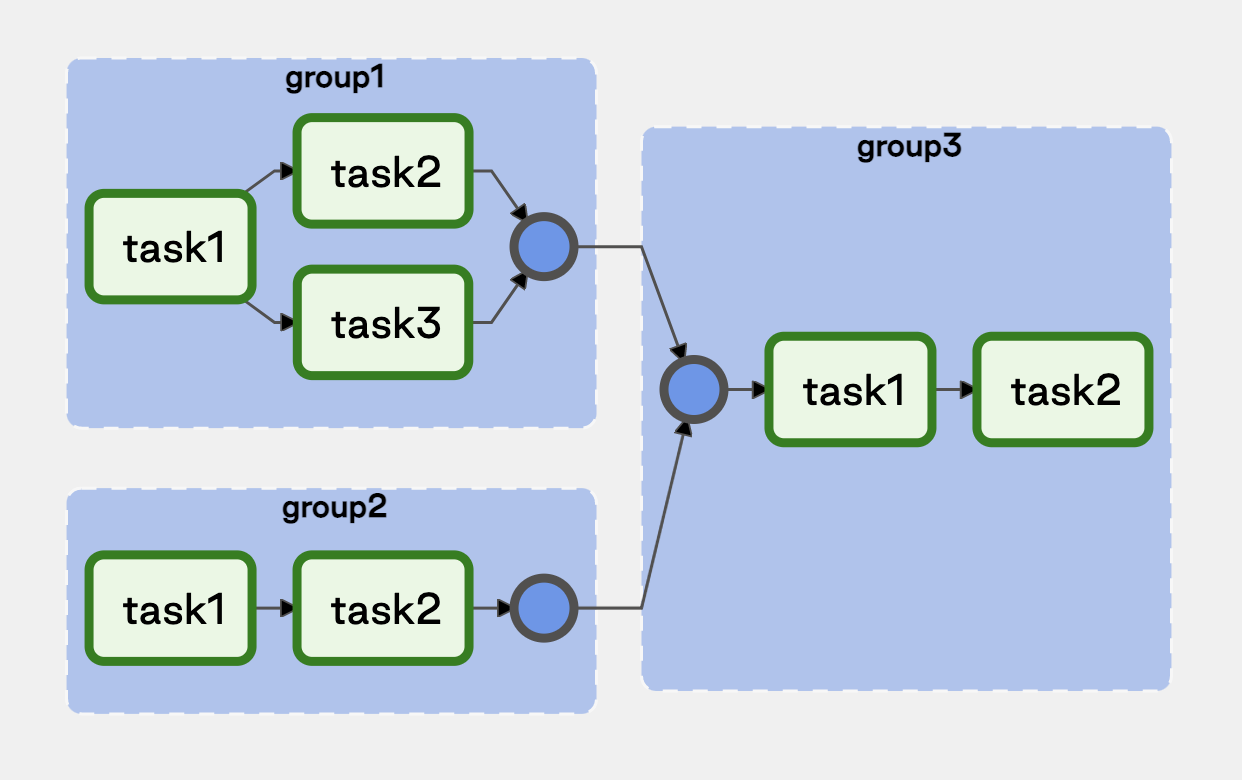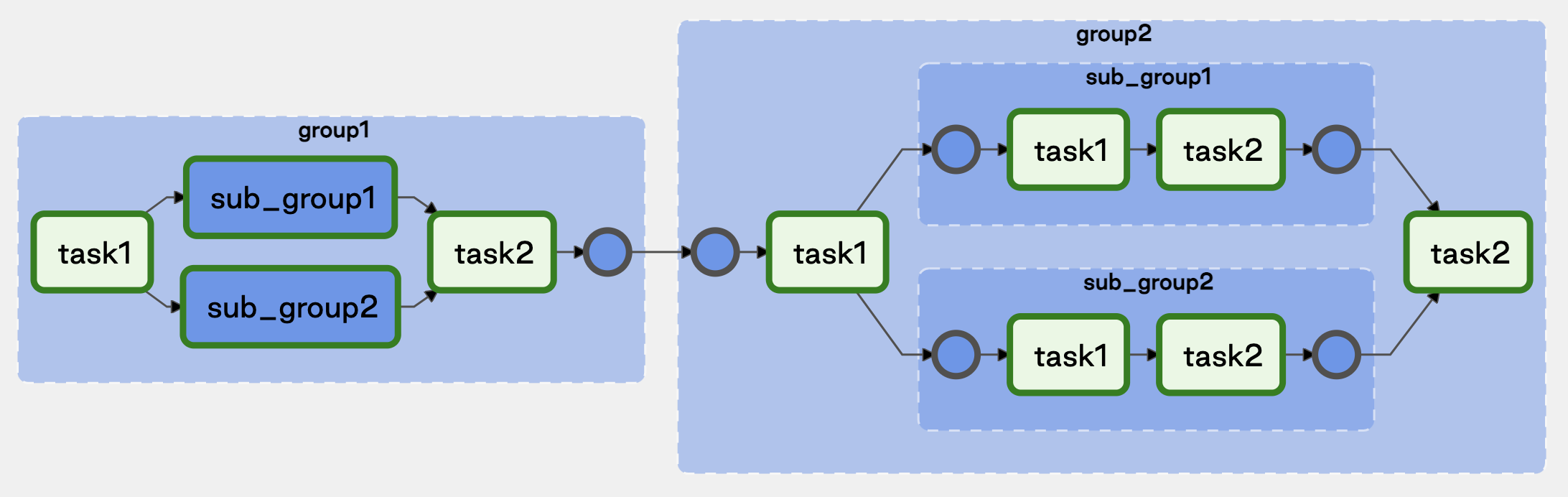Use task groups to organize tasks in the Airflow UI DAG graph view.

In this guide, you'll learn how to create task groups and review some example DAGs that demonstrate their scalability.

## Assumed knowledge​

To get the most out of this guide, you should have an understanding of:

To use task groups, run the following import statement:

``from airflow.utils.task_group import TaskGroup``

For your first example, you'll instantiate a task group using a `with` statement and provide a `group_id`. Inside your task group, you'll define your two tasks, `t1` and `t2`, and their respective dependencies.

You can use dependency operators (`<<` and `>>`) in task groups in the same way that you can with individual tasks. Dependencies applied to a task group are applied across its tasks. In the following code, you'll add additional dependencies to `t0` and `t3` to the task group, which automatically applies the same dependencies across `t1` and `t2`:

``t0 = EmptyOperator(task_id='start')# Start task group definitionwith TaskGroup(group_id='group1') as tg1:    t1 = EmptyOperator(task_id='task1')    t2 = EmptyOperator(task_id='task2')    t1 >> t2# End task group definition    t3 = EmptyOperator(task_id='end')# Set task group's (tg1) dependenciest0 >> tg1 >> t3``

In the Airflow UI, blue highlighting is used to identify tasks and task groups. When you click and expand `group1`, blue circles identify the task group dependencies. The task immediately to the right of the first blue circle (`t1`) gets the group's upstream dependencies and the task immediately to the left (`t2`) of the last blue circle gets the group's downstream dependencies. The task group dependencies are shown in the following animation:When your task is within a task group, your callable `task_id` is the `task_id` prefixed with the `group_id`. For example, `group_id.task_id`. This ensures the task_id is unique across the DAG. It is important that you use this format when calling specific tasks with XCOM passing or branching operator decisions.

## Use the task group decorator​

To use the decorator, add `@task_group` before a Python function which calls the functions of tasks that should go in the task group. For example:

``@task_group(group_id="tasks")def my_independent_tasks():    task_a()    task_b()    task_c()``

This function creates a task group with three independent tasks that are defined elsewhere in the DAG.

You can also create a task group of dependent tasks. For example:

``@task_group(group_id="tasks")def my_dependent_tasks():    return task_a(task_b(task_c()))``

The following DAG shows a full example implementation of the task group decorator, including passing data between tasks before and after the task group:

``import jsonfrom airflow.decorators import dag, task, task_groupimport pendulum@dag(schedule=None, start_date=pendulum.datetime(2021, 1, 1, tz="UTC"), catchup=False)def task_group_example():    @task(task_id='extract', retries=2)    def extract_data():        data_string = '{"1001": 301.27, "1002": 433.21, "1003": 502.22}'        order_data_dict = json.loads(data_string)        return order_data_dict    @task()    def transform_sum(order_data_dict: dict):        total_order_value = 0        for value in order_data_dict.values():            total_order_value += value        return {"total_order_value": total_order_value}    @task()    def transform_avg(order_data_dict: dict):        total_order_value = 0        for value in order_data_dict.values():            total_order_value += value            avg_order_value = total_order_value / len(order_data_dict)        return {"avg_order_value": avg_order_value}    @task_group    def transform_values(order_data_dict):        return {'avg': transform_avg(order_data_dict), 'total': transform_sum(order_data_dict)}    @task()    def load(order_values: dict):        print(f"Total order value is: {order_values['total']['total_order_value']:.2f} and average order value is: {order_values['avg']['avg_order_value']:.2f}")    load(transform_values(extract_data()))    task_group_example = task_group_example()``

The resulting DAG looks similar to this image:There are a few things to consider when using the task group decorator:

• If downstream tasks require the output of tasks that are in the task group decorator, then the task group function must return a result. In the previous example, a dictionary with two values was returned, one from each of the tasks in the task group, that are then passed to the downstream `load()` task.
• If your task group function returns an output, you can call the function from your DAG with the TaskFlow API. If your task group function does not return any output, you must use the bitshift operators (`<<` or `>>`) to define dependencies to the task group.

## Generate task groups dynamically at runtime​

As of Airflow 2.5, you can use dynamic task mapping with the `@task_group` decorator to dynamically map over task groups. The following DAG shows how you can dynamically maps over a task group with different inputs for a given parameter.

``from airflow import DAGfrom airflow.decorators import task_group, taskfrom pendulum import datetimewith DAG(    dag_id="task_group_mapping_example",    start_date=datetime(2022, 12, 1),    schedule=None,    catchup=False,):    # creating a task group using the decorator with the dynamic input my_num    @task_group(        group_id="group1"    )    def tg1(my_num):        @task        def print_num(num):            return num                @task        def add_42(num):            return num + 42                print_num(my_num) >> add_42(my_num)    # a downstream task to print out resulting XComs    @task    def pull_xcom(**context):        pulled_xcom = context["ti"].xcom_pull(            # reference a task in a task group with task_group_id.task_id            task_ids=['group1.add_42'],             # only pull xcom from specific mapped task group instances (2.5 feature)            map_indexes=[2, 3],             key="return_value"        )        # will print out a list of results from map index 2 and 3 of the add_42 task         print(pulled_xcom)    # creating 6 mapped task group instances of the task group group1 (2.5 feature)    tg1_object = tg1.expand(my_num=[19, 23, 42, 8, 7, 108])    # setting dependencies    tg1_object >> pull_xcom()``

This DAG dynamically maps over the task group `group1` with different inputs for the `my_num` parameter. 6 mapped task group instances are created, one for each input. Within each mapped task group instance two tasks will run using that instances' value for `my_num` as an input. The `pull_xcom()` task downstream of the dynamically mapped task group shows how to access a specific XCom value from a list of mapped task group instances (`map_indexes`).

In the following example, the third task group generated in the loop has a foreign key constraint on both previously generated task groups (first and second iteration of the loop), so you'll want to process it last. To do this, you'll create an empty list and append your task Group objects as they are generated. Using this list, you can reference the task groups and define their dependencies to each other:

``groups = []for g_id in range(1,4):    tg_id = f'group{g_id}'    with TaskGroup(group_id=tg_id) as tg1:        t1 = EmptyOperator(task_id='task1')        t2 = EmptyOperator(task_id='task2')        t1 >> t2        if tg_id == 'group1':            t3 = EmptyOperator(task_id='task3')            t1 >> t3                        groups.append(tg1)[groups , groups] >> groups``

The following image shows how these task groups appear in the Airflow UI:In the previous example, you added an additional task to `group1` based on your `group_id`. This demonstrated that even though you're creating task groups in a loop to take advantage of patterns, you can still introduce variations to the pattern while avoiding code redundancies introduced by building each task group definition manually.

``groups = []for g_id in range(1,3):    with TaskGroup(group_id=f'group{g_id}') as tg1:        t1 = EmptyOperator(task_id='task1')        t2 = EmptyOperator(task_id='task2')        sub_groups = []        for s_id in range(1,3):            with TaskGroup(group_id=f'sub_group{s_id}') as tg2:                st1 = EmptyOperator(task_id='task1')                st2 = EmptyOperator(task_id='task2')                st1 >> st2                sub_groups.append(tg2)        t1 >> sub_groups >> t2        groups.append(tg1)groups >> groups``